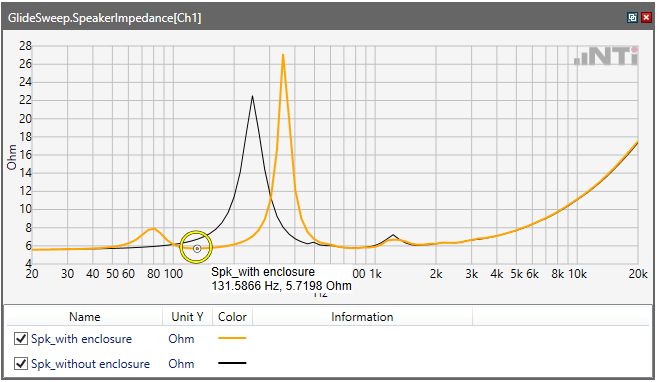Start a new topic

## How to measure/calculate Fb (Resonance frequency with sound box)?

As question above, Please help us set up HW & SW to measure/calculate Fb (Resonance frequency with sound box).

We found "Resonance frequency" calculation on Fx-control software, but I think that's only F0 (Resonance frequency of driver, without soundbox)

We are using Fx100 with Fx-SIP module.

Many thanks!

Hello Gregor Schmidle,

I received your FX-Control project file.

That's all I need. It's really helpful to me.

Thank you very much!

Hello Hoang Nguyen Thanh,

thanks for the clarification. I now know what you mean and I do have a solution for it.

1. From the impedance response, take a "Curve Subrange" where you expect fb to be located.  (in my example, below this is between 40Hz and 100Hz

2. From this subrange, we can calcuate the frequency where the lowest value of the subrange is located. This is consequently fbBest Regards,

Gregor

1 person likes this

Hi Gregor Schmidle,

i'm afraid of you're slightly confused.

With driver: Impedance curve only has 1 peak.....F0 is Frequency at this peak (Black curve)

With loudspeaker [driver+sound box]: Impedance curve has 2 peaks...... Fb is a frequency at minimum point between the 2 peaks. (point in yellow circle on orange curve)

But, I don't know formula to calculate Fb from impedance curve :)

Another way, Fb is frequency at point that has phase angle =0. So, Could we add "phase curve" and "Impedance curve" on one graph?

Thanks!

Hello Hoang Nguyen Thanh,

I am not particular familiar with the paramter fb.

fb     ...    Resonance frequency with sound box

f0     ...    Resonance frequency of driver, with sound box

I would assume that these are the frequencies where f0 and fb are located like this:So f0 would be the value calculated by the "Resonance frequency" calculation item with the input of the black curve,

and fb would be the value calculated by the "Resonance frequency" calculation item with the input of the yellow curve,

Best Regards,

Gregor

Hi Gregor Schmidle,

For example as picture below,

In my opinion, the default "Resonance frequency" calculation item on RT-control only shows the highest peak.

But with the loudspeaker enclosure, I want to know how to calculate Fb.

Could you explain in more detail!Thanks!

Hello Hoang Nguyen Thanh,

The "Resonance frequency" calculation item is able to calculate a resonance frequency out of any impedance response.

When the impedance reponse is measured of the driver only, the calculated frequency is the responance frequency of that driver.

When the impedance response is measured of the loudspeaker enclosure, the calculated frequency is the resonance frequency of that loudspeaker.

The loudspeaker can have multiple resonance frequencies.  (Bass reflex, Woofer, Mid-range, Tweeter)

You can calculate an individual resonance frequency of an indiviudal component by limiting the frequency Scope in the calculation item.

I hope this helps.

Let me know if I can be of further assistance.

Best Regards,

Gregor

Gregor Schmidle

Product Manager

NTi Audio AG, Im alten Riet 102,
9494 Schaan, Liechtenstein, Europe